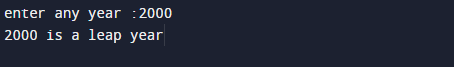# C Program to Check Leap Year

In this tutorial you will learn about the C Program to Check Leap Year and its application with practical example.

In this tutorial, you will learn to find given year is a leap year or not.

## Prerequisites

Before starting with this tutorial we assume that you are best aware of the following C programming topics:

• C Operators
• Conditional statement.
• Basic input output .

## Example:

So logic behind to check whether a year is leap or not is:
Logic of Leap Year:
If the given value is completely divisible by 4, 100 and 400 then it is a leap year.
If the given value is divisible by 4 but not  by 100 then it is a leap year.

Logic for  not a Leap Year:
If the given value is not divisible by 4 then it is not a leap year
If the given value is divisible by 4 and 100 but not divisible by 400 then it is not a leap year.

For example,

• 1221 is not a leap year.
• 2000 is a leap year.
• 1890 is not  a leap year.

## Program to Check given year is a Leap Year or not.

Here fist we take a year from user and with help of logical operator(&& and ,|| or) we will check multiple condition in a single if statement and get the required result.

## OutputIn our program, we have first declared and initialized a set variables required in the program.

• year =taking year value from user.

First of all take a value from user to check whether a year is leap or not is,
then we check if the given value is completely divisible by 4, 100 and 400 then it is a leap year.
If the given value is divisible by 4 but not  by 100 then it is a leap year.

In this tutorial we have learn about the C Program to Check Leap Year and its application with practical example. I hope you will like this tutorial.